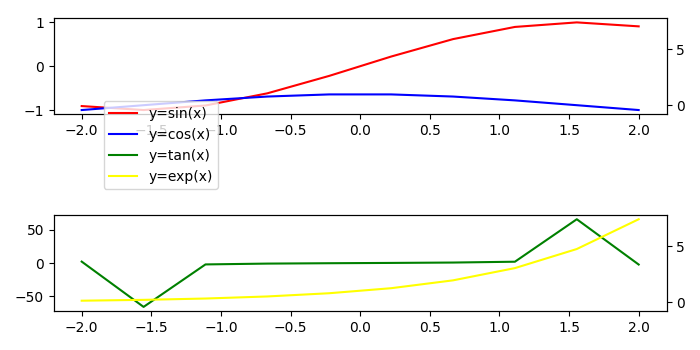# How to share secondary Y-axis between subplots in Matplotlib?

To share secondary Y-axis between subplots in matplotlib, we can take the following steps −

• Create for data points.

• Add a subplot to the current figure, with nrows=2, ncols=1, at index=1 (ax0)

• Using twinx() method, create a twin of axes with a shared X-axis but independent Y-axis (ax1).

• Add a subplot to the current figure, with nrows=2, ncols=1 at index=2 (ax2)

• Using twinx() method, create a twin of axes with a shared X-axis but independent Y-axis (ax3).

• Using get_shared_y_axes() method, return a reference to the shared axes Grouper object for Yaxis.

• Create curves c1, c2, c3 and c4 with different colors, x and y data points.

• To move the legend box to the upper left of the figure, use the legend method with bbox_to_anchor.

• To display the figure, use show() method.

## Example

import numpy as np
from matplotlib import pyplot as plt
plt.rcParams["figure.figsize"] = [7.00, 3.50]
plt.rcParams["figure.autolayout"] = True
x = np.linspace(-2, 2, 10)
ax0 = plt.subplot(211)
ax1 = ax0.twinx() # Create a twin of Axes with a shared x-axis but independent y-axis.
ax2 = plt.subplot(212)
ax3 = ax2.twinx() # Create a twin of Axes with a shared x-axis but independent y-axis.
ax1.get_shared_y_axes().join(ax1, ax3)
c1, = ax0.plot(x, np.sin(x), c='red')
c2, = ax1.plot(x, np.cos(x), c='blue')
c3, = ax2.plot(x, np.tan(x), c='green')
c4, = ax3.plot(x, np.exp(x), c='yellow')
plt.legend([c1, c2, c3, c4], ["y=sin(x)", "y=cos(x)", "y=tan(x)", "y=exp(x)"],
loc = "upper left", bbox_to_anchor=(.070, 2.25))
plt.show()

## OutputUpdated on: 07-May-2021

3K+ Views